# mshap_plots

## Introduction and Data Creation

This vignette will demonstrate how to customize and add on to the default plots created by the plotting functions available in the {mshap} package. Since branding and layout are important in industry, it is often necessary to customize a chart well beyond the default settings. First, we will set up out R libraries.

# Load Libraries
library(mshap)
library(ggplot2)
#> Warning: package 'ggplot2' was built under R version 4.0.2
library(dplyr)
#> Warning: package 'dplyr' was built under R version 4.0.2
#>
#> Attaching package: 'dplyr'
#> The following objects are masked from 'package:stats':
#>
#>     filter, lag
#> The following objects are masked from 'package:base':
#>
#>     intersect, setdiff, setequal, union

We will assume for the purpose of this exercise a two-part model that predicts the total amount of jet fuel we will need for a single aircraft in the upcoming year. The first part of this model predicts the number of flights the aircraft will make in the year, and the second part of this model predicts the average fuel consumption per flight. Both models will have the following covariates:

• age: age of aircraft, in years
• prop_domestic: the number of domestic (within country) flights that the aircraft has done over the past year out of its total number of flights
• model: our fleet is made up of two models, model A (0) and model B (1)
• maintain: the number of hours spent maintaining the aircraft over the past year

We will generate random values for these covariates and then generate fake mSHAP values for the final (nonexistent) model so that we can use these in plotting.

set.seed(18)

dat <- data.frame(
age = runif(1000, min = 0, max = 20),
prop_domestic = runif(1000),
model = sample(c(0, 1), 1000, replace = TRUE),
maintain = rexp(1000, .01) + 200
)

shap <- data.frame(
age = rexp(1000, 1/dat$age) * (-1)^(rbinom(1000, 1, dat$prop_domestic)),
prop_domestic = -200 * rnorm(100, dat$prop_domestic, 0.02) + 100, model = ifelse(dat$model == 0, rnorm(1000, -50, 30), rnorm(1000, 50, 30)),
maintain = (rnorm(1000, dat\$maintain, 100) - 400) * 0.2
)

## Summary Plot

The first type of plot we will cover is the summary plot, which is generated by a call to mshap::summary_plot(). In its most simple form, the plot is as follows:

summary_plot(
variable_values = dat,
shap_values = shap
)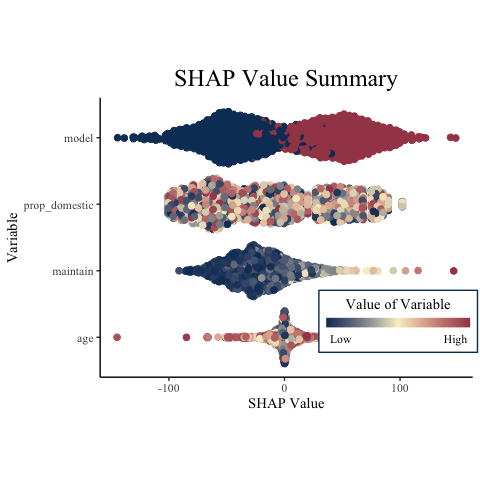Note that the function automatically orders the variables from the most important to least important SHAP values (as measured by average absolute value of the SHAP value).

There are several things that we might want to change about this plot. The first and most obvious is that the legend is covering some of our data. We can use the legend.position argument to change it to the bottom of the plot.

summary_plot(
variable_values = dat,
shap_values = shap,
legend.position = "bottom"
)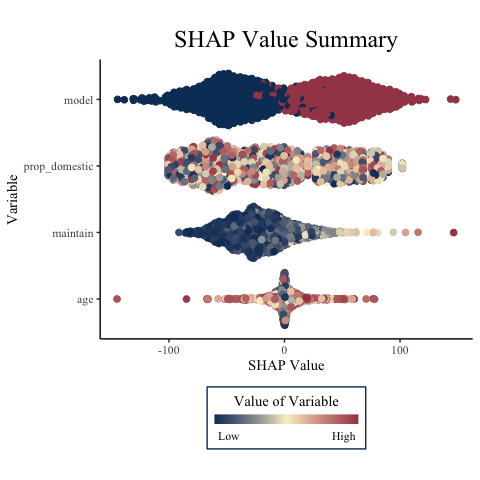Now suppose that we aren’t very happy with the names of the variables, as we want to present this plot to people who do not code and might be unfamiliar with a variable name format like prop_domestic. Using the names argument, we can specify different names for our data, just ensuring that they are in the same order as both variables_values and shap_values.

summary_plot(
variable_values = dat,
shap_values = shap,
legend.position = "bottom",
names = c("Age", "% Domestic", "Model", "Maintenence Hours")
)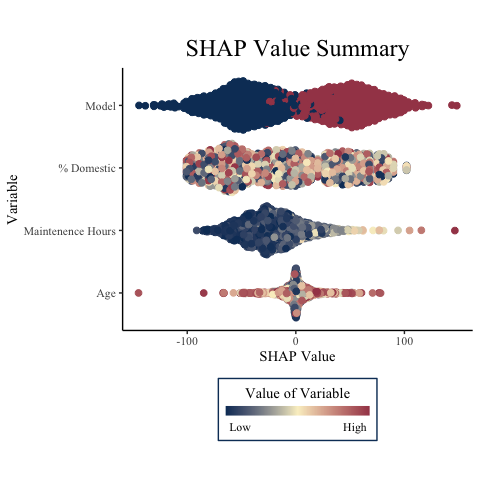Finally, we may wish to adjust the theme by changing the colors of the plot and making all the text be in Arial font. Also, we can specify the title by using the title parameter.

summary_plot(
variable_values = dat,
shap_values = shap,
legend.position = "bottom",
names = c("Age", "% Domestic", "Model", "Maintenence Hours"),
colorscale = c("blue", "purple", "red"),
font_family = "Arial",
title = "A Custom Title"
)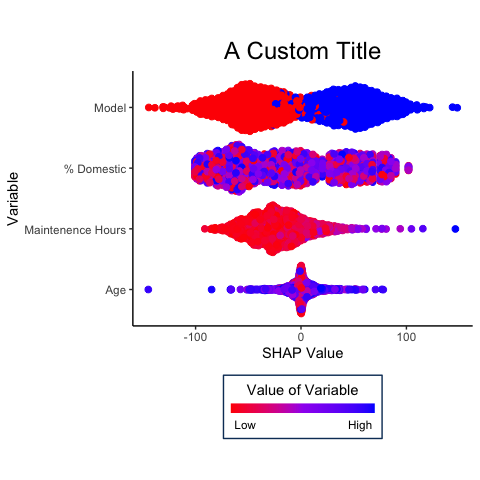## Observation Plot

The other function used for plotting in {mshap} is observation_plot(). This function takes a single row of variable values and SHAP values to create a plot showing why the model made the prediction it did for that value.

For this, we will need an expected value of our model, which we will arbitrarily set to 1,000. Normally the expected value that will be used is returned from the mshap() function.

expected_value <- 1000

With this expected value, we can now create the most basic plot.

observation_plot(
variable_values = dat[1,],
shap_values = shap[1,],
expected_value = expected_value
)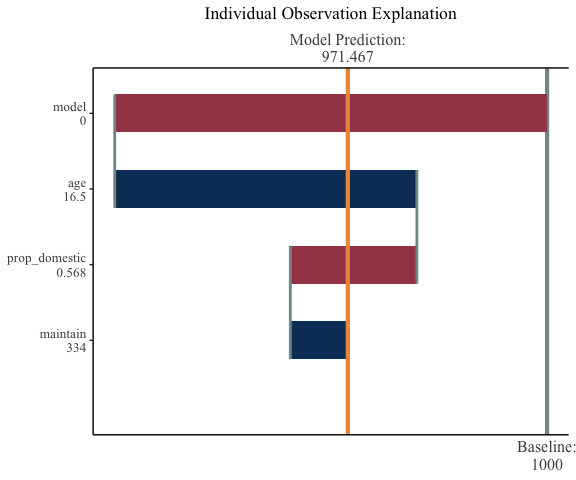From this plot, we can see that both the model and the proportion of domestic flights push the prediction down, while the maintenance and the age cause the prediction to be pushed up, and it ultimately settles around 971.

Some of the arguments to observation_plot() are similar to those of summary_plot(). First, we will reset the names, change the font, and add a title.

observation_plot(
variable_values = dat[1,],
shap_values = shap[1,],
expected_value = expected_value,
names = c("Age", "% Domestic", "Model", "Maintenence Hours"),
font_family = "Arial",
title = "A Custom Title"
)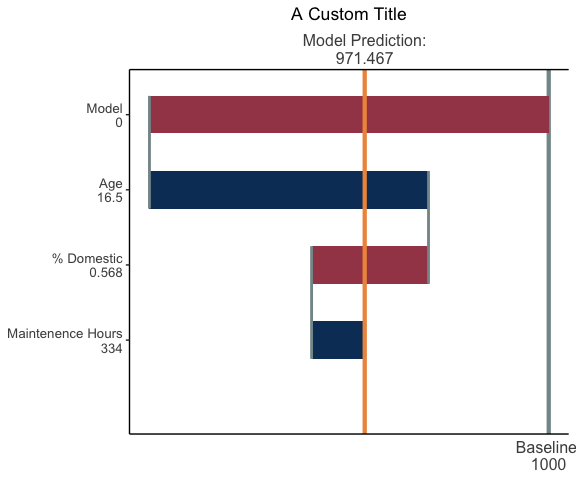If we would prefer to show “A” as the model instead of 0, we can use the following code:

observation_plot(
variable_values = dat[1,] %>% mutate(model = ifelse(model == 0, "A", "B")),
shap_values = shap[1,],
expected_value = expected_value,
names = c("Age", "% Domestic", "Model", "Maintenence Hours"),
font_family = "Arial",
title = "A Custom Title"
)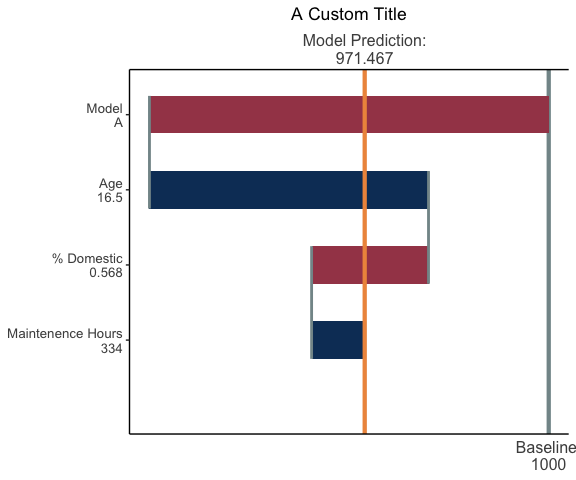Finally, we can change the colors on this plot to match the brighter red and blue shown earlier. The argument fill_colors specifies the fill (the negative fill first, then the positive fill), while the connect_color controls the color of the connecting line between the SHAP value bars. Also, the color of the expected model output line can be changed with expected_color and the color of the predicted value line can be changed with predicted_color.

observation_plot(
variable_values = dat[1,] %>% mutate(model = ifelse(model == 0, "A", "B")),
shap_values = shap[1,],
expected_value = expected_value,
names = c("Age", "% Domestic", "Model", "Maintenence Hours"),
font_family = "Arial",
title = "A Custom Title",
fill_colors = c("red", "blue"),
connect_color = "black",
expected_color = "purple",
predicted_color = "yellow"
)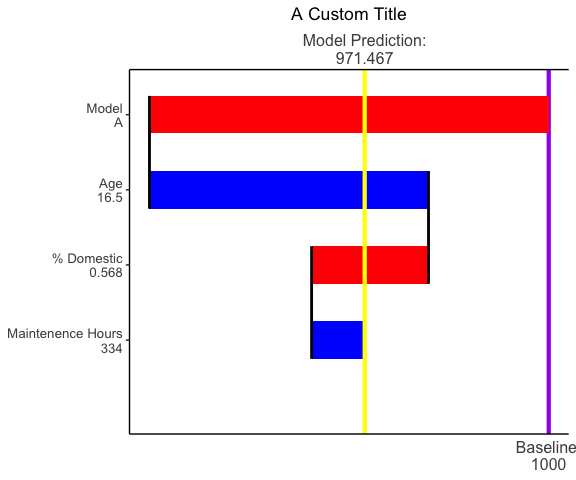The functions demonstrated above return {ggplot2} objects, which means that additional elements or layers can be added on top of the returned plots. For instance, if we want to change the background and panel color on one of the summary plots above, we can add a theme() layer with the specified background color.

summary_plot(
variable_values = dat,
shap_values = shap,
legend.position = "bottom",
names = c("Age", "% Domestic", "Model", "Maintenence Hours")
) +
theme(
plot.background = element_rect(fill = "grey"),
panel.background = element_rect(fill = "lightgrey")
)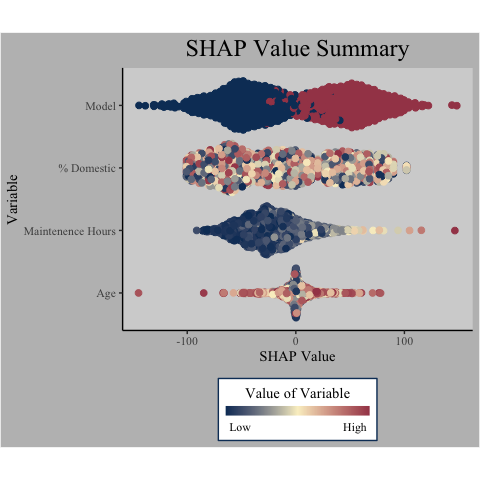We can also add text and labels and other objects to our plots. In the following code, we add a label to one of the SHAP value bars.
A few IMPORTANT notes: - We have to specify a numeric y, which must be done manually since the strings are converted to factors in the back end. Counting goes from the bottom to the top. - There is a call to ggplot::coord_flip() inside mshap::observation_plot() which means that sometimes when adding new objects, the x and y aesthetics must be reverse of what you are expecting.

observation_plot(
variable_values = dat[1,] %>% mutate(model = ifelse(model == 0, "A", "B")),
shap_values = shap[1,],
expected_value = expected_value,
names = c("Age", "% Domestic", "Model", "Maintenence Hours"),
font_family = "Arial",
title = "A Custom Title"
) +
geom_label(
aes(y = 950, x = 4, label = "This is a really big bar!"),
color = "#FFFFFF",
fill = NA
)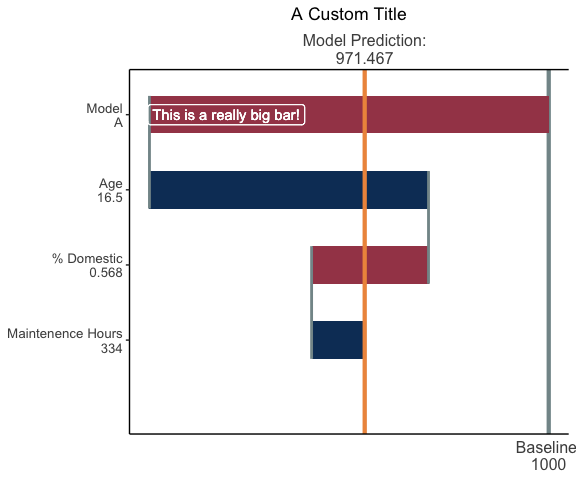## Conclusion

Hopefully these plotting tools will be beneficial in your use of {mSHAP}, and that you are able to customize the plots as needed. If you have a customization need that is not currently possible, feel free to submit a pull request!